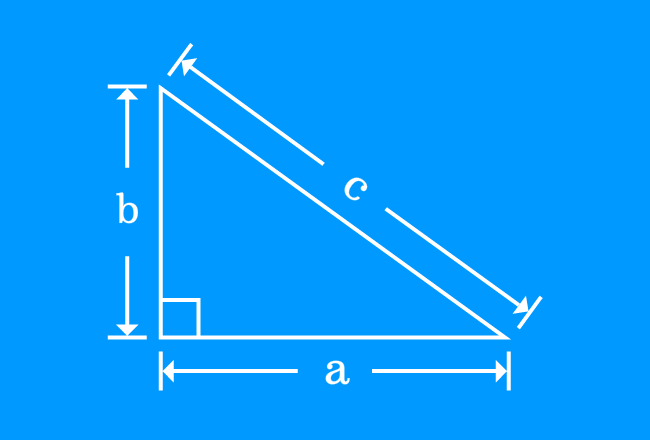# Pythagorean Theorem

The square of length of hypotenuse equals to the sum of squares of opposite and adjacent sides of a right triangle is called the Pythagorean Theorem.

## Introduction

A Greek Mathematician Pythagoras identified the direct geometrical relationship between the sides of a right triangle.He observed that the square of length of hypotenuse of a right triangle is exactly equal to the sum of squares of lengths of opposite and adjacent sides of the same triangle.

#### Example

If $a$, $b$ and $c$ are lengths of opposite side, adjacent side and hypotenuse of a right triangle, then direct relation between them can be expressed in mathematical form as follows.

$\large c^2 \,=\, a^2+b^2$

### Proof

Learn how to derive the Pythagorean theorem in algebraic form by the geometrical system.

Latest Math Topics
Jun 26, 2023
Jun 23, 2023

Latest Math Problems
Jul 01, 2023
Jun 25, 2023
###### Math Questions

The math problems with solutions to learn how to solve a problem.

Learn solutions

Practice now

###### Math Videos

The math videos tutorials with visual graphics to learn every concept.

Watch now

###### Subscribe us

Get the latest math updates from the Math Doubts by subscribing us.